# Fractals and Epicycles

There is no bilaterally-symmetrical, nor eccentrically-periodic curve used in any branch of astrophysics or observational astronomy which could not be smoothly plotted as the resultant motion of a point turning within a constellation of epicycles, finite in number, revolving around a fixed deferent.

Norwood Russell Hanson, “The Mathematical Power of Epicyclical Astronomy”

A friend recently showed me this image…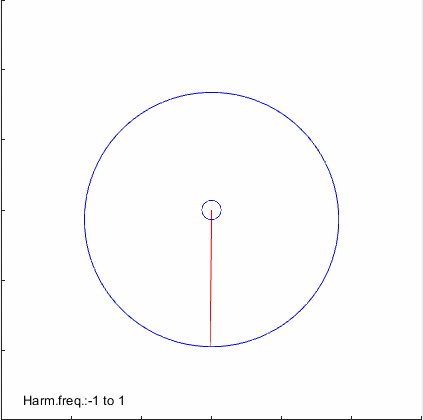…and thus I was drawn into the world of epicycles and fractals.

Epicycles were first used by the Greeks to reconcile observational data of the motions of the planets with the theory that all bodies orbit the Earth in perfect circles. It was found that epicycles allowed astronomers to retain their belief in perfectly circular orbits, as well as the centrality of Earth. The cost of this, however, was a system with many adjustable parameters (as many parameters as there were epicycles).

There’s a somewhat common trope about adding on endless epicycles to a theory, the idea being that by being overly flexible and accommodating of data you lose epistemic credibility. This happens to fit perfectly with my most recent posts on model selection and overfitting! The epicycle view of the solar system is one that is able to explain virtually any observational data. (There’s a pretty cool reason for this that has to do with the properties of Fourier series, but I won’t go into it.) The cost of this is a massive model with many parameters. The heliocentric model of the solar system, coupled with the Newtonian theory of gravity, turns out to be able to match all the same data with far fewer adjustable parameters. So by all of the model selection criteria we went over, it makes sense to switch over from one to the other.

Of course, it is not the case that we should have been able to tell a priori that an epicycle model of the planets’ motions was a bad idea. “Every planet orbits Earth on at most one epicycle”, for instance, is a perfectly reasonable scientific hypothesis… it just so happened that it didn’t fit the data. And adding epicycles to improve the fit to data is also not bad scientific practice, so long as you aren’t ignoring other equally good models with fewer parameters.)

Okay, enough blabbing. On to the pretty pictures! I was fascinated by the Hilbert curve drawn above, so I decided to write up a program of my own that generates custom fractal images from epicycles. Here are some gifs I created for your enjoyment:

## Negative doubling of angular velocity

(Each arm rotates in the opposite direction of the previous arm, and at twice its angular velocity. The length of each arm is half that of the previous.)## Trebling of angular velocity## Negative trebling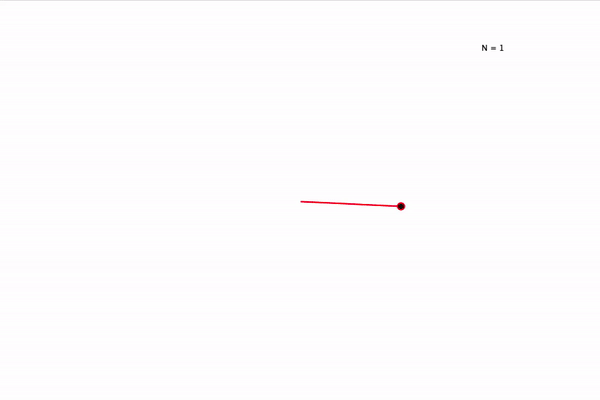Here’s a still frame of the final product for N = 20 epicycles: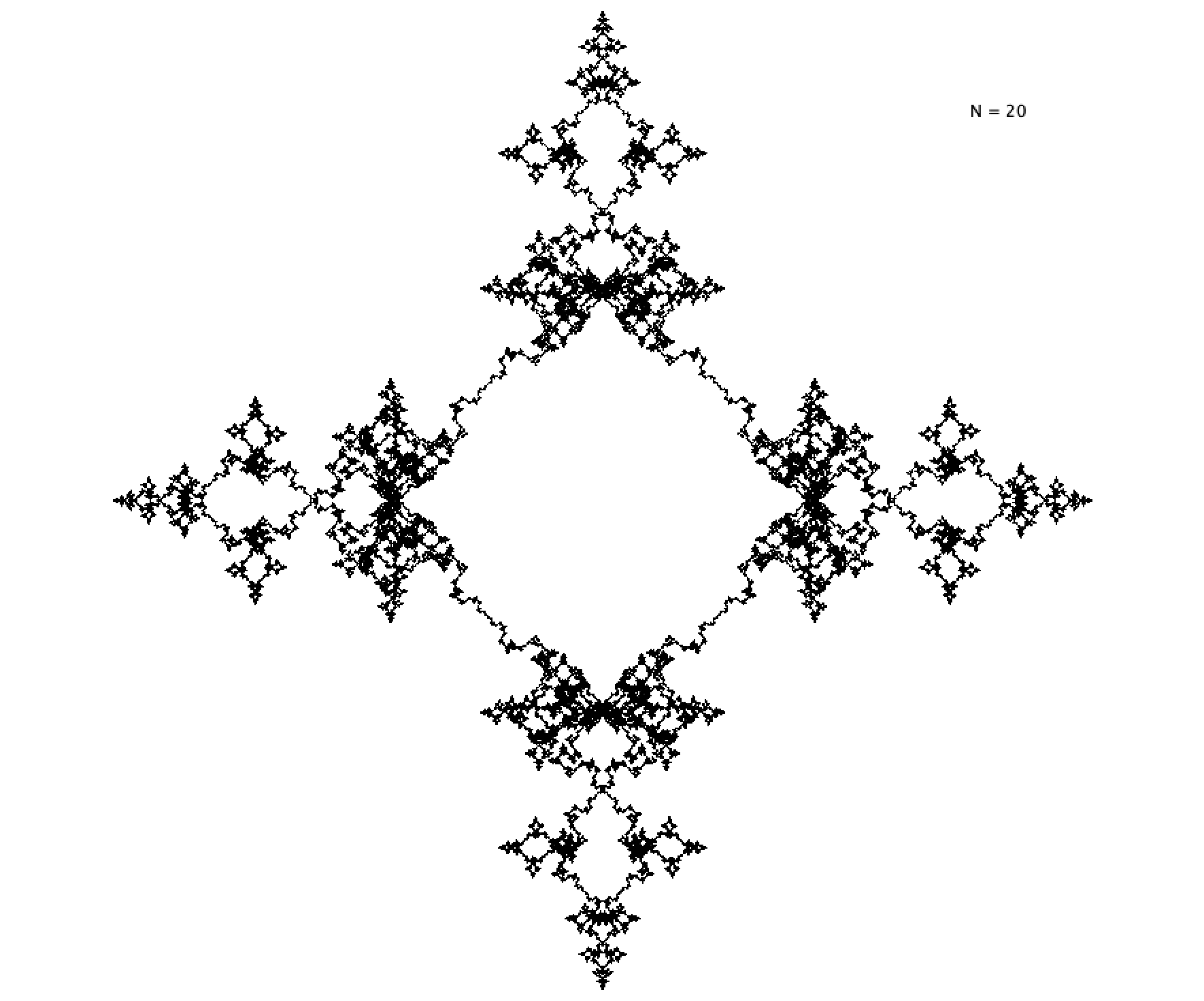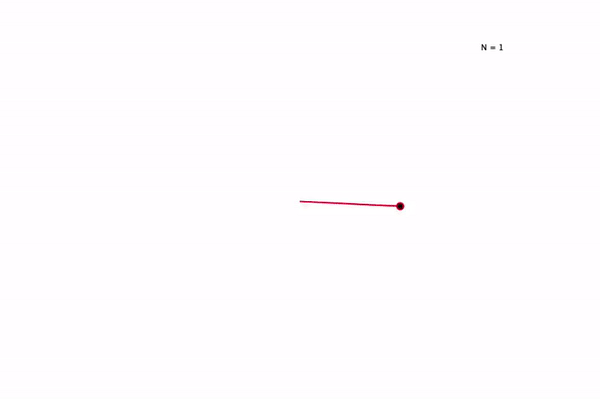## ωn ~ (n+1) 2n

(or, the Fractal Frog)## ωn ~ n, rn ~ 1/n## ωn ~ n, constant rn## ωn ~ 2n, rn ~ 1/n2And here’s a still frame of N = 20: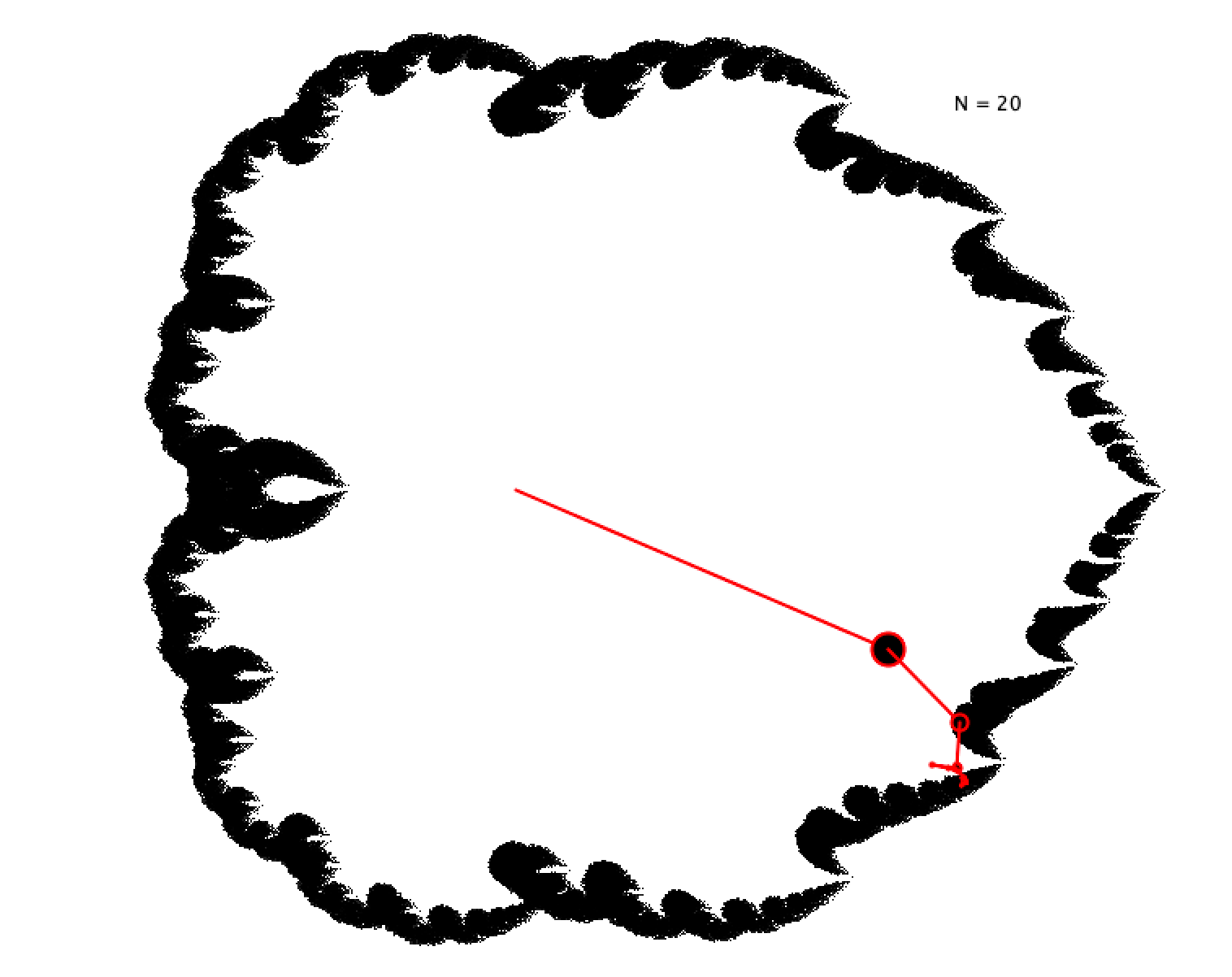(All animations were built using Processing.py, which I highly recommend for quick and easy construction of visualizations.)

# How to Learn From Data, Part 2: Evaluating Models

I ended the last post by saying that the solution to the problem of overfitting all relied on the concept of a model. So what is a model? Quite simply, a model is just a set of functions, each of which is a candidate for the true distribution that generates the data.

Why use a model? If we think about a model as just a set of functions, this seems kinda abstract and strange. But I think it can be made intuitive, and that in fact we reason in terms of models all the time. Think about how physicists formulate their theories. Laws of physics have two parts: First they specify the types of functional relationships there are in the world. And second, they specify the value of particular parameters in those functions. This is exactly how a model works!

Take Newton’s theory of gravity. The fundamental claim of this theory is that there is a certain functional relationship between the quantities a (the acceleration of an object), M (the mass of a nearby object), and r (the distance between the two objects): a ~ M/r2. To make this relationship precise, we need to include some constant parameter G that says exactly what this proportionality is: a = GM/r2.

So we start off with a huge set of probability distributions over observations of the accelerations of particles (one for each value of G), and then we gather data to see which value of G is most likely to be right. Einstein’s theory of gravity was another different model, specifying a different functional relationship between a, M, and r and involving its own parameters. So to compare Einstein’s theory of gravity to Newton’s is to compare different models of the phenomenon of gravitation.

When you start to think about it, the concept of a model is an enormous one. Models are ubiquitous, you can’t escape from them. Many fancy methods in machine learning are really just glorified model selection. For instance, a neural network is a model: the architecture of the network specifies a particular functional relationship between the input and the output, and the strengths of connections between the different neurons are the parameters of the model.

So. What are some methods for assessing predictive accuracy of models? The first one we’ll talk about is a super beautiful and clever technique called…

## Cross Validation

The fundamental idea of cross validation is that you can approximate how well a model will do on the next data point by evaluating how the model would have done at predicting a subset of your data, if all it had access to was the rest of your data.

I’ll illustrate this with a simple example. Suppose you have a data set of four points, each of which is a tuple of two real numbers. Your two models are T1: that the relationship is linear, and T2: that the relationship is quadratic.

What we can do is go through each data point, selecting each in order, train the model on the non-selected data points, and then evaluate how well this trained model fits the selected data point. (By training the model, I mean something like “finding the curve within the model that best fits the non-selected data points.”) Here’s a sketch of what this looks like: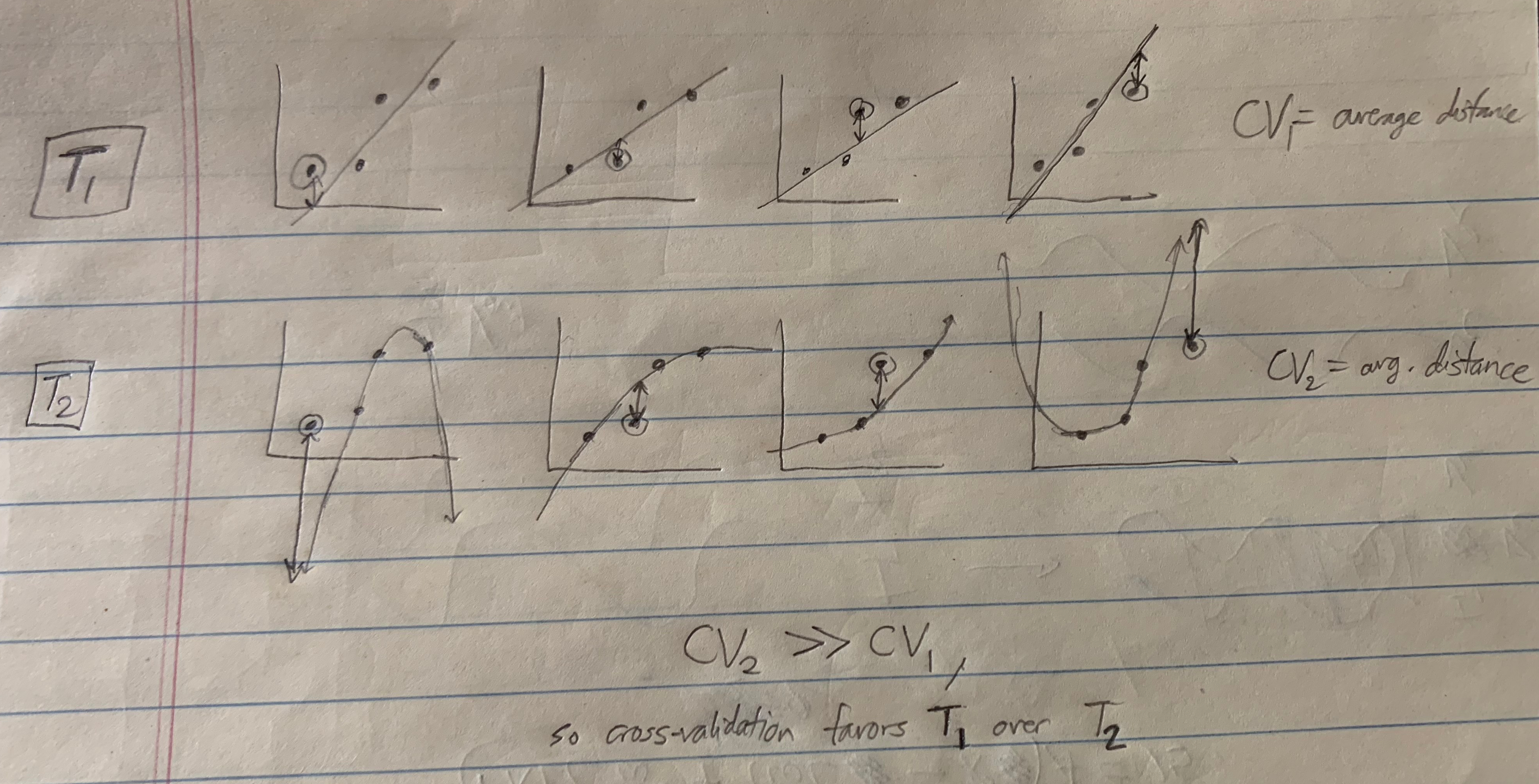There are a bunch of different ways of doing cross validation. We just left out one data point at a time, but we could have left out two points at a time, or three, or any k less than the total number of points N. This is called leave-k-out cross validation. If we partition our data by choosing a fraction of it for testing, then we get what’s called n-fold cross validation (where 1/n is the fraction of the data that is isolated for testing).

We also have some other choices besides how to partition the data. For instance, we can choose to train our model via a Likelihoodist procedure or a Bayesian procedure. And we can also choose to test our model in various ways, by using different metrics for evaluating the distance between the testing set and the trained model. In leave-one-out cross validation (LOOCV), a pretty popular method, both the training and testing procedures are Likelihoodist.

Now, there’s a practical problem with cross-validation, which is that it can take a long time to compute. If we do LOOCV with N data points and look at all ways of leaving out one data point, then we end up doing N optimization procedures (one for each time you train your model), each of which can be pretty slow. But putting aside practical issues, for thinking about theoretical rationality, this is a super beautiful technique.

Next we’ll move on to…

## Bayesian Model Selection!

We had Bayesian procedures for evaluating individual functions. Now we can go full Bayes and apply it to models! For each model, we assess the posterior probability of the model given the data using Bayes’ rule as usual:$Pr(M | D) = \frac{Pr(D | M)}{Pr(D)} Pr(M)$

Now… what is the prior Pr(M)? Again, it’s unspecified. Maybe there’s a good prior that solves overfitting, but it’s not immediately obvious what exactly it would be.

But there’s something really cool here. In this equation, there’s a solution to overfitting that pops up not out of the prior, but out of the LIKELIHOOD! I explain this here. The general idea is that models that are prone to overfitting get that way because their space of functions is very large. But if the space of functions is large, then the average prior on each function in the model must be small. So larger models have, on average, smaller values of the term Pr(f | M), and correspondingly (via$Pr(D | M) = \sum_{f \in M} Pr(D | f, M) Pr(f | M)$) get a weaker update from evidence.

So even without saying anything about the prior, we can see that Bayesian model selection provides a potential antidote to overfitting. But just like before, we have the practical problem that computing Pr(M | D) is in general very hard. Usually evaluating Pr(D | M) involves calculating a really complicated many-dimensional integral, and calculating many-dimensional integrals can be very computationally expensive.

Might we be able to find some simple unbiased estimator of the posterior Pr(M | D)? It turns out that yes, we can. Take another look at the equation above.$Pr(M | D) = \frac{Pr(D | M)}{Pr(D)} Pr(M)$

Since$Pr(D)$ is a constant across models, we can ignore it when comparing models. So for our purposes, we can attempt to maximize the equation:$Pr(D | M) Pr(M) = \sum_{f \in M} Pr(D | f, M) Pr(f | M) Pr(M)$

If we assume that P(D | f) is twice differentiable in the model parameters and that Pr(f) behaves roughly linearly around the maximum likelihood function, we can approximate this as:$Pr(D | M) Pr(M) \approx \frac {Pr(D | f^*(D))} {\sqrt{N}}^k Pr(M)$

f* is the maximum likelihood function within the model, and k is the the number of parameters of the model (e.g. k = 2 for a linear model and k = 3 for a quadratic model).

\We can make this a little neater by taking a logarithm and defining a new quantity: the Bayesian Information Criterion.$argmax_M [ Pr(M | D) ] \\~\\ = argmax_M [ Pr(D | M) Pr(M) ] \\~\\ \approx argmax_M [ \frac {Pr(D | f^*(D))} {\sqrt{N}}^k Pr(M)] \\~\\ = argmax_M [ \log(Pr(M)) + \log (Pr(D | f^*(D)) - \frac{k}{2} \log(N) ] \\~\\ = argmin_M [ BIC - \log Pr(M) ]$$BIC = \frac{k}{2} log(N) - \log \left( Pr(D | f^*(D)) \right)$

Thus, to maximize the posterior probability of a model M is roughly the same as to minimize the quantity BIC – log Pr(M).

If we assume that our prior over models is constant (that is, that all models are equally probable at the outset), then we just minimize BIC.

## Bayesian Information Criterion

Notice how incredibly simple this expression is to evaluate! If all we’re doing is minimizing BIC, then we only need to find the maximum likelihood function f*(D), assess the value of Pr(D | f*), and then penalize this quantity with the factor k/2 log(N)! This penalty scales in proportion to the model complexity, and thus helps us avoid overfitting.

We can think about this as a way to make precise the claims above about Bayesian Model Selection penalizing overfitting in the likelihood. Remember that minimizing BIC made sense when we assumed a uniform prior over models (and therefore when our prior doesn’t penalize overfitting). So even when we don’t penalize overfitting in the prior, we still end up getting a penalty! This penalty must come from the likelihood.

Some more interesting facts about BIC:

• It is approximately equal to the minimum description length criterion (which I haven’t discussed here)
• It is only valid when N >> k. So it’s not good for small data sets or large models.
• It the truth is contained within your set of models, then BIC will select the truth with probability 1 as N goes to infinity.

Okay, stepping back. We sort of have two distinct clusters of approaches to model selection. There’s Bayesian Model Selection and the Bayesian Information Criterion, and then there’s Cross Validation. Both sound really nice. But how do they compare? It turns out that in general they give qualitatively different answers. And in general, the answers you get using cross validation tend to be more predictively accurate that those that you get from BIC/BMS.

A natural question to ask at this point is: BIC was a nice simple approximation to BMS. Is there a corresponding nice simple approximation to cross validation? Well first we must ask: which cross validation? Remember that there was a plethora of different forms of cross validation, each corresponding to slightly different criterion for evaluating fits. We can’t assume that all these methods give the same answer.

Let’s choose leave-one-out cross validation. It turns out that yes, there is a nice approximation that is asymptotically equivalent to LOOCV! This is called the Akaike information criterion.

## Akaike Information Criterion

First, let’s define AIC:$AIC = k - \log \left( Pr(D | f^*(D)) \right)$

Like always, k is the number of parameters in M and f* is chosen by the Likelihoodist approach described in the last post.

What you get by minimizing this quantity is asymptotically equivalent to what you get by doing leave-one-out cross validation. Compare this to BIC:$BIC = \frac{k}{2} \log (N) - \log \left( Pr(D | f^*(D) ) \right)$

There’s a qualitative difference in how the parameter penalty is weighted! BIC is going to have a WAY higher complexity penalty than AIC. This means that AIC should in general choose less simple models than BIC.

Now, we’ve already seen one reason why AIC is good: it’s a super simple approximation of LOOCV and LOOCV is good. But wait, there’s more! AIC can be derived as an unbiased estimator of the Kullback-Leibler divergence DKL.

What is DKL? It’s a measure of the information theoretic distance of a model from truth. For instance, if the true generating distribution for a process is f, and our model of this process is g, then DKL tells us how much information we lose by using g to represent f. Formally, DKL is:$D_{KL}(f, g) = \int { f(x) \log \left(\frac{f(x)} {g(x)} \right) dx }$

Notice that to actually calculate this quantity, you have to already know what the true distribution f is. So that’s unfeasible. BUT! AIC gives you an unbiased estimate of its value! (The proof of this is complicated, and makes the assumptions that N >> k, and that the model is not far from the truth.)

Thus, AIC gives you an unbiased estimate of which model is closest to the truth. Even if the truth is not actually contained within your set of models, what you’ll end up with is the closest model to it. And correspondingly, you’ll end up getting a theory of the process that is very similar to how the process actually works.

There’s another version of AIC that works well for smaller sample sizes and larger models called AICc.$AICc = AIC + \frac {k (k + 1)} {N - (k + 1)}$

And interestingly, AIC can be derived as an approximation to Bayesian Model Selection with a particular prior over models. (Remember earlier when we showed that$argmax_M \left( Pr(M | D) \right) \approx argmin_M \left( BIC - \log(Pr(M)) \right)$? Well, just set$\log Pr(M) = BIC - AIC = k \left( \frac{1}{2} \log N - 1 \right)$, and you get the desired result.) Interestingly, this prior ends up rewarding theories for having lots of parameters! This looks pretty bad… it seems like AIC is what you get when you take Bayesian Model Selection and then try to choose a prior that favors overfitting theories. But the practical use and theoretical virtues of AIC warrant, in my opinion, taking a closer look at what’s going on here. Perhaps what’s going on is that the likelihood term Pr(D | M) is actually doing too much to avoid overfitting, so in the end what we need in our prior is one that avoids underfitting! Regardless, we can think of AIC as specifying the unique good prior on models that optimizes for predictive accuracy.

There’s a lot more to be said from here. This is only a short wade into the waters of statistical inference and model selection. But I think this is a good place to stop, and I hope that I’ve given you a sense of the philosophical richness of the various different frameworks for inference I’ve presented here.

# How to Learn From Data, Part I: Evaluating Simple Hypotheses

Here’s a general question of great importance: How should we adjust our model of the world in response to data? This post is a tour of a few of the big ideas that humans have come up with to address this question. I find learning about this topic immensely rewarding, and so I shall attempt to share it! What I love most of all is thinking about how all of this applies to real world examples of inference from data, so I encourage you to constantly apply what I say to situations that you find personally interesting.

First of all, we want a formal description of what we mean by data. Let’s describe our data quite simply as a set:$D = \{(x_1, y_1), (x_2, y_2),..., (x_N, y_N)\}$, where each$x_i$ is a value that you choose (your independent variable) and each$y_i$ is the resulting value that you measure (the dependent variable). Each of these variables can be pretty much anything – real numbers, n-tuples of real numbers, integers, colors, whatever you want. The goal here is to embrace generality, so as to have a framework that applies to many kinds of inference.

Now, suppose that you have a certain theory about the underlying relationship between the x variables and the y variables. This theory might take the form of a simple function:$T: y = f(x)$ We interpret T as making a prediction of a particular value of the dependent variable for each value of the independent variable. Maybe the data is the temperatures of regions at various altitudes, and our theory T says that one over the temperature (1/T) is some particular linear function of the altitude.

What we want is a notion of how good of a theory T is, given our data. Intuitively, we might think about doing this by simply assessing the distance between each data point$y_n$ and the predicted value of y at that point:$f(x_n)$, using some metric, then adding them all up. But there are a whole bunch of distance metrics available to us. Which one should we use? Perhaps the taxicab measure comes to mind ($\sum_{n=1}^N {|y_n - f(x_n)|}$), or the sum of the squares of the differences ($SOS = \sum_{n=1}^N {(y_n - f(x_n))^2}$). We want a good theoretical justification for why any one of these metrics should be preferred over any other, since in general they lead to different conclusions. We’ll see just such justifications shortly. Keep the equation for SOS in mind, as it will turn up repeatedly ahead.

Now here’s a problem: If we want a probabilistic evaluation of T, then we have to face the fact that it makes a deterministic prediction. Our theory seems to predict with 100% probability that at$x_n$ the observed$y_n$ will be precisely$f(x_n)$. If it’s even slightly off of this, then our theory will be probabilistically disconfirmed to zero.

We can solve this problem by modifying our theory T to not just be a theory of the data, but also a theory of error. In other words, we expect that the value we get will not be exactly the predicted value, and give some account of how on average observation should differ from theory.$T: y = f(x) + \epsilon$, where$\epsilon$ is some random variable drawn from a probability distribution$P_E$.

This error distribution can be whatever we please – Gaussian, exponential, Poisson, whatever. For simplicity let’s say that we know the error is normal (drawn from a Gaussian distribution) with a known standard deviation σ.$T: y = f(x) + \epsilon, \epsilon \sim N(0, \sigma)$

A note on notation here:$\epsilon \sim N(\mu, \sigma)$ denotes a random variable drawn from a Gaussian distribution centered around$\mu$ with a standard deviation of$\sigma$.

This gives us a sensible notion of the probability of obtaining some value of y from a chosen x given the theory T.$Pr(y | x, T) \sim N(f(x), \sigma) \\~\\ Pr(y | x, T) = \frac{1}{\sqrt{2 \pi \sigma^2}} e^{-\frac{1}{2 \sigma^2} (y - f(x))^2}$

Nice! This is a crucial step towards figuring out how to evaluate theories: we’ve developed a formalism for describing precisely how likely a data point is, given a particular theory (which, remember, so far is just a function from values of independent variables to values of dependent variables, coupled with a theory of how the error in your observations works).

Let’s try to extend this to our entire data set D. We want to assess the probability of the particular values of the dependent variables, given the chosen values of the dependent variables and the function. We’ll call this the probability of D given f.$Pr(D | f) = Pr(x_1, y_1, x_2, y_2,..., x_N, y_N | T) \\~\\ Pr(D | f) = Pr(y_1, y_2,...,y_N | x_1, x_2,..., x_N, T)$

(It’s okay to move the values of the independent variable x across the conditional bar because of our assumption that we know beforehand what values x will take on.)

But now we run into a problem: we can’t really do anything with this expression without knowing how the data points depend upon each other. We’d like to break it into individual terms, each of which can be evaluated by the above expression for$Pr(y | x, T)$. But that would require an assumption that our data points are independent. In general, we cannot grant this assumption. But what we can do is expand our theory once more to include a theory of the dependencies in our data points. For simplicity of explication, let’s proceed with the assumption that each of our observations is independent of the others. Said another way, we assume that given T,$x_n$ screens off all other variables from$y_n$). Combined with our assumption of normal error, this gives us a nice simple reduction.$Pr(D | f) = Pr(y_1, y_2,...,y_N | x_1, x_2,..., x_N, T) \\~\\ Pr(D | f) = Pr(y_1 | x_1, T) Pr(y_2 | x_2, T) ... Pr(y_N | x_N, T) \\~\\ Pr(D | f) = \prod_{n=1}^N { \frac{1}{\sqrt{2 \pi \sigma^2}} e^{-\frac{1}{2 \sigma^2} (y_n - f(x_n))^2} } \\~\\ Pr(D | f) = (2 \pi \sigma^2)^{-N/2} e^{-\frac{1}{2 \sigma^2} \sum_{n=1}^N {(y_n - f(x_n))^2}} \\~\\ Pr(D | f) = (2 \pi \sigma^2)^{-N/2} e^{-\frac{SOS(f, D)}{2 \sigma^2} }$

We see an interesting connection arise here between Pr(D | f) and the sum of squares evaluation of fit. More will be said of this in just a moment.

But first, let’s take a step back. Our ultimate goal here is to find a criterion for theory evaluation. And here we’ve arrived at an expression that looks like it might be right for that role! Perhaps we want to say that our criterion for evaluating theories is just maximization of Pr(D | f). This makes some intuitive sense… a good theory is one which predicts the observed data. If general relativity or quantum mechanics predicted that the sun should orbit the earth, or that atoms should be unstable, then we wouldn’t be very in favor of it.

And so we get the Likelihoodism Thesis!

Likelihoodism: The best theory f* given some data D is that which maximizes Pr(D | f). Formally:$f^*(D) = argmax_f [ Pr(D | f) ]$

Now, since logarithms are monotonic, we can express this in a more familiar form:$argmax_f [Pr(D | F)] = argmax_f \left[ e^{-\frac{SOS(f, D)}{2 \sigma^2} } \right] = argmax_f \left[-\frac{SOS(f, D)}{2 \sigma^2} \right] = argmin_f [ SOS(f, D) ]$

Thus the best theory according to Likelihoodism is that which minimizes the sum of squares! People minimize sums of squares all the time, and most of the time they don’t realize that it can be derived from the Likelihoodism Thesis and the assumptions of Gaussian error and independent data. If our assumptions had been different, then we would have found a different expression, and SOS might no longer be appropriate! For instance, the taxicab metric arises as the correct metric if we assume exponential error rather than normal error. I encourage you to see why this is for yourself. It’s a fun exercise to see how different assumptions about the data give rise to different metrics for evaluating the distance between theory and observation. In general, you should now be able to take any theory of error and assess what the proper metric for “degree of fit of theory to data” is, assuming that degree of fit is evaluated by maximizing Pr(D | f).

Now, there’s a well-known problem with the Likelihoodism thesis. This is that the function f that minimizes SOS(f, D) for some data D is typically going to be a ridiculously overcomplicated function that perfectly fits each data points, but does terribly on future data. The function will miss the underlying trend in the data for the noise in the observations, and as a result fail to get predictive accuracy.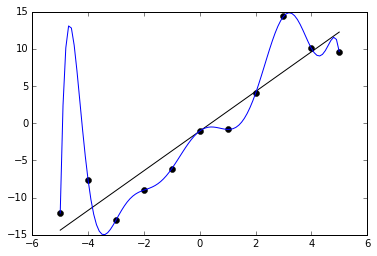This is the problem of overfitting. Likelihoodism will always prefer theories that overfit to those that don’t, and as a result will fail to identify underlying patterns in data and do a terrible job at predicting future data.

How do we solve this? We need a replacement for the Likelihoodism thesis. Here’s a suggestion: we might say that the problem stems from the fact that the Likelihoodist procedure recommends us to find a function that makes the data most probable, rather than finding a function that is made most probable by the data. From this suggestion we get the Bayesian thesis:

Bayesianism: The best theory f given some data is that which maximizes Pr(f | D).$f^*(D) = argmax_f [ Pr(f | D) ]$

Now, what is this Pr(f | D) term? It’s an expression that we haven’t seen so far. How do we evaluate it? We simply use the theorem that the thesis is named for: Bayes’ rule!$Pr(f | D) = \frac{Pr(D | f)}{Pr(D)} Pr(f)$

This famous theorem is a simple deductive consequence of the probability axioms and the definition of conditional probability. And it happens to be exactly what we need here. Notice that the right-hand side consists of the term Pr(D | f), which we already know how to calculate. And since we’re ultimately only interested in varying f to find the function that maximizes this expression, we can ignore the constant term in the denominator.$argmax_f [ Pr(f | D) ] = argmax_f [ Pr(D | f) Pr(f) ] \\~\\ argmax_f [ Pr(f | D) ] = argmax_f [ \log Pr(D | f) + \log Pr(f) ] \\~\\ argmax_f [ Pr(f | D) ] = argmax_f [ -\frac{SOS(f, D)}{2 \sigma^2} + \log Pr(f) ] \\~\\ argmax_f [ Pr(f | D) ] = argmin_f [ SOS(f, D) - 2 \sigma^2 \log Pr(f) ]$

The last steps we get just by substituting in what we calculated before. The 2σ² in the first term comes from the fact that the exponent of our Gaussian is (y – f(x))² / 2σ². We ignored it before because it was just a constant, but since we now have another term in the expression being maximized, we have to keep track of it again.

Notice that what we get is just what we had initially (a sum of squares) plus an additional term involving a mysterious Pr(f). What is this Pr(f)? It’s our prior distribution over theories. Because of the negative sign in front of the second term, a larger value of Pr(f) gives a smaller value for the expression that we are minimizing. Similarly, the more closely our function follows the data, the smaller the SOS term becomes. So what we get is a balance between fitting the data and having a high prior. A theory that fits the data perfectly can still end up with a bad evaluation, as long as it has a low enough prior. And a theory that fits the data poorly can end up with a great evaluation if it has a high enough prior.

(Those that are familiar with machine learning might notice that this feels similar to regularization. They’re right! It turns out that different regularization techniques just end up corresponding to different Bayesian priors! L2 regularization corresponds to a Gaussian prior over parameters, and L1 regularization corresponds to an exponential prior over parameters. And so on. Different prior assumptions about the process generating the data lead naturally to different regularization techniques.)

What this means is that if we are trying to solve overfitting, then the Bayesianism thesis provides us a hopeful ray of light. Maybe we can find some perfect prior Pr(f) that penalizes complex overfitting theories just enough to give us the best prediction. But unsurprisingly, finding such a prior is no easy matter. And importantly, the Bayesian thesis as I’ve described it gives us no explicit prescription for what this prior should be, making it insufficient for a full account of inference. What Bayesianism does is open up a space of possible methods of theory evaluation, inside which (we hope) there might be a solution to our problem.

Okay, let’s take another big step back. How do we progress from here? Well, let’s think for a moment about what our ultimate goal is. We want to say that overfitting is bad, and that therefore some priors are better than others insofar as they prevent overfitting. But what is the standard we’re using to determine that overfitting is bad? What’s wrong with an overfitting theory?

Here’s a plausible answer: The problem with overfitting is that while it maximizes descriptive accuracy, it leads to poor predictive accuracy! I.e., theories that overfit do a great job at describing past data, but tend to do a poor job of matching future data.

Let’s take this idea and run with it. Perhaps all that we truly care about in theory selection is predictive accuracy. I’ll give a name to this thesis:

Predictivism: The ultimate standard for theory evaluation is predictive accuracy. I.e., the best theory is that which does the best at predicting future data.

Predictivism is a different type of thesis than Bayesianism and Likelihoodism. While each of those gave precise prescriptions for how to calculate the best theory, Predictivism does not. If we want an explicit algorithm for computing the best theory, then we need to say what exactly “predictive accuracy” means. This means that as far as we know so far, Predictivism could be equivalent to Likelihoodism or to Bayesianism. It all depends on what method we end up using to determine what theory has the greatest predictive accuracy. Of course, we have good reasons to suspect that Likelihoodism is not identical to Predictivism (Likelihoodism overfits, and we suspect that Predictivism will not) or to Bayesianism (Bayesianism does not prescribe a particular prior, so it gives no unique prescription for the best theory). But what exactly Predictivism does say depends greatly on how exactly we formalize the notion of predictive accuracy.

Another difference between Predictivism and Likelihoodism/Bayesianism is that predictive accuracy is about future data. While it’s in principle possible to find an analytic solution to P(D | f) or P(f | D), Predictivism seems to require us to compute terms involving future data! But these are impossible to specify, because we don’t know what the future data will be!

Can we somehow approximate what the best theory is according to Predictivism, by taking our best guess at what the future data will be? Maybe we can try to take the expected value of something like$Pr(y_{N+1} | x_{N+1}, T, D)$, where$(x_{N+1}, y_{N+1})$ is a future data point. But there are two big problems with this.

First, in taking an expected value, you must use the distribution of outcomes given by your own theory. But then your evaluation picks up an inevitable bias! You can’t rely on your own theories to assess how good your theories are.

And second, by the assumptions we’ve included in our theories so far, the future data will be independent of the past data! This is a really big problem. We want to use our past data to give some sense of how well we’ll do on future data. But if our data is independent, then our assessment of how well T will do on the next data point will have to be independent of the past data as well! After all, no matter what value$y_{N+1}$ has,$Pr(y_{N+1} | x_{N+1}, T, D) = Pr(y_{N+1} | x_{N+1}, T).$

So is there any way out of this? Surprisingly, yes! If we get creative, we can find ways to approximate this probability with some minimal assumptions about the data. These methods all end up relying on a new concept that we haven’t yet discussed: the concept of a model. The next post will go into more detail on how this works. Stay tuned!

# The Match ProblemThis puzzle has been popping up on my Facebook feed recently. Try to see how high you can get before reading on!

(…)

Now, would you be surprised if I told you that with a little interpretive freedom and creativity, you can get numbers larger than the number of atoms in the observable universe? How about if I told you that you can get to numbers large enough that they break set theory? Let me demonstrate for you.

You can move the bottom match in the 5 up to make it a 9. Then you can rotate the bottom left vertical match in the 0 to make it a nine. This gives 998.Can we do better? Sure! Take the top and bottom matches in the central zero and move them to squeeze in another one, giving you 51,118.So far we’ve been working purely in base 10. Let’s try to do better by moving to a more exotic number system.

Hexadecimal is a base-16 number system, the digits of which are written as 0, 1, 2, 3, 4, 5, 6, 7, 8, 9, A, B, C, D, E, and F. For instance, we can construct F88:F88 is only 3976, which is not better than our best so far (51,118). If we’re allowed to interpret a square of matches as a D instead of a zero, we can do slightly better (though to do so we have to be allowed to throw out a toothpick, or place it directly on top of another one):FDB is 4059, which is still not better than our current best. To get better, we need to get more clever.

# Exponentiation!

Our first strategy is just to shift two matches in the first digit down so as to make it into a 9:We can interpret this as 98 = 43,046,721. This is our best so far! But we can do better by applying Knuth’s up-arrow notation.This is 518, which is almost 3.8 trillion, 100 thousand times better than 98! But we’re not done yet! If we use a caret (^) to represent exponentiation, we can get even higher!5118 is 5.4 nonillion, a number with 31 decimal digits long. We could try to do better than this by squeezing in a caret between the 5 and the 1, making 5118 (a number with 83 decimal digits) but this becomes pretty messy and gross.

Alright, so we got up to 83 digit long numbers. Can we get any better? Yep!

# Tetration

Tetration is the level above exponentiation. The nth tetration of a is defined as follows:Just as multiplication is repeated addition and exponentiation is repeated multiplication, tetration is repeated exponentiation! Now we get into numbers whose size is truly impossible to grasp. Let’s shift the top and bottom matches on the middle 0:We can write this equivalently as:How big is this number? There’s really no meaningful way to describe it. We’ve gone far beyond any quantities that can be made physical sense of, like the number of cubic nanometers in the universe, which is a measly 10107. But we’re not done yet!

# Busy Beavers!

The busy beaver numbers are a sequence of numbers that arise from the properties of Turing machines. Here’s a brief description: The nth busy beaver number B(N) is the greatest number of ones that a finitely-running N state Turing machine can print on a tape which starts all zero.

Did you think tetration is big? Well, busy beaver numbers are unimaginably larger. In fact, the busy beaver sequence grows larger than any computable function! There’s a neat proof of this that involves the uncomputability of the halting problem. To skim over it, it can be shown that were we to have an upper bound on the busy beaver sequence, then we could find a deterministic algorithm for solving the halting problem in a finite amount of time, which we know is impossible. And if any computable function F(N) grew faster than B(N), then we could find an upper bound on the busy beaver sequence. Thus, it must be the case that no computable function grows as fast as B(N)!

We can exploit the absurd growth rate of the busy beaver sequence if we are allowed the interpretative freedom of assuming parentheses, so that Bn = B(n).Let’s think for a minute about how large B(118) must be. So far, the only values of n for which B(n) is known are B(1), B(2), B(3), and B(4). After this the values grow out of control. A lower bound on B(6) is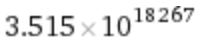. For B(7), we have as a lower bound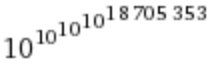. B(8) almost certainly beats our current record. And B(118) is unthinkably large.

We can get even higher than the busy beaver numbers with the maximum shifts function S(N), defined as the number of steps that the longest-finitely-running N state Turing machine takes before halting. This function is guaranteed to be larger than B(N) for all N. Using S(N), and taking the same liberties as above with respect to parentheses, we can get an insanely high value:This is S(1118), and while it’s undoubtedly larger than B(118), there’s no way to get any intuitive grasp on how much larger. But wait, there’s more! We can get even larger by recursively nesting a busy beaver function within a maximum shifts function:We interpret this as S(B(9)). Why is this larger than S(1118)? Well, B(9) is some enormous number, certainly larger than 1118, so S(B(9)) is certainly greater than S(1118).

Now, are we finally done? Have we reached the peak yet? No! It’s time for the largest solution of them all.

The reason that the Busy Beaver numbers and Maximum Shift function are so big is because of the uncomputability of the halting problem. But if we consider Turing machines that have an oracle for the halting problem (call these meta Turing machines), we get a new meta-halting problem: when do these meta Turing machines halt? From the meta-halting problem comes an associated new sequence of Busy Beaver numbers, which grows uncomputable faster than the original Busy Beaver sequence. Then we can equip Turing machines with an oracle for the meta-halting problem, generating a meta-meta-Busy Beaver sequence.

Thus we get a hierarchy of Busy Beaver functions, which, following the notation used by Scott Aaronson here, can be described with Bn(x). Each Bn grows uncomputably faster than the previous Bn-1. There’s a similar hierarchy for the maximum shifts function, and each S_n is going to be an upper bound on each Sn-1.

So we can exploit this hierarchy to create an unimaginably large number (whose actual value is almost certainly independent of the axioms of set theory): Move around the top and bottom matches on the 0 to give S a subscript of 11. Then we get the 11th-up-in-the-hierarchy maximum shifts function S11 applied to 118: S11(118).It’s a little gross-looking, but I think it works! I challenge anybody to try to come up with a better solution. 🙂

# Gödel’s Second Incompleteness Theorem: Explained in Words of Only One Syllable

Somebody recently referred me to a 1994 paper by George Boolos in which he writes out a description of Gödel’s Second Incompleteness Theorem, using only words of one syllable. I love it so much that I’m going to copy the whole thing here in this post. Enjoy!

First of all, when I say “proved”, what I will mean is “proved with the aid of the whole of math”. Now then: two plus two is four, as you well know. And, of course, it can be proved that two plus two is four (proved, that is, with the aid of the whole of math, as I said, though in the case of two plus two, of course we do not need the whole of math to prove that it is four). And, as may not be quite so clear, it can be proved that it can be proved that two plus two is four, as well. And it can be proved that it can be proved that it can be proved that two plus two is four. And so on. In fact, if a claim can be proved, then it can be proved that the claim can be proved. And that too can be proved.

Now, two plus two is not five. And it can be proved that two plus two is not five. And it can be proved that it can be proved that two plus two is not five, and so on.

Thus: it can be proved that two plus two is not five. Can it be proved as well that two plus two is five? It would be a real blow to math, to say the least, if it could. If it could be proved that two plus two is five, then it could be proved that five is not five, and then there would be no claim that could not be proved, and math would be a lot of bunk.

So, we now want to ask, can it be proved that it can’t be proved that two plus two is five? Here’s the shock: no, it can’t. Or, to hedge a bit: if it can be proved that it can’t be proved that two plus two is five, then it can be proved as well that two plus two is five, and math is a lot of bunk. In fact, if math is not a lot of bunk, then no claim of the form “claim X can’t be proved” can be proved.

So, if math is not a lot of bunk, then, though it can’t be proved that two plus two is five, it can’t be proved that it can’t be proved that two plus two is five.

By the way, in case you’d like to know: yes, it can be proved that if it can be proved that it can’t be proved that two plus two is five, then it can be proved that two plus two is five.

George Boolos, Mind, Vol. 103, January 1994, pp. 1 – 3